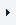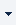LOGINREGISTER (FREE TRIAL)myTOMLAB
 ProductsTOMLAB Base ModuleTOMLAB /MINOSTOMLAB /NPSOLTOMLAB /DNOPTTOMLAB /SNOPTTOMLAB /SOLTOMLAB /CGOTOMLAB /CPLEXTOMLAB /GUROBITOMLAB /MINLPTOMLAB /MIPNLPTOMLAB /MISQPSolversTOMLAB /PENSDPTOMLAB /PENBMITOMLAB /KNITROTOMLAB /OQNLPTOMLAB /PROPTTOMLAB /NLPQLTOMLAB /LGOTOMLAB /LGO-MINLPTOMLAB /GPTOMLAB /GENOTOMLAB /MADTOMLAB /MIDACO

# TOMLAB /MISQP

TOMLAB /MISQP integrates The MathWorks' MATLAB and the MISQP and NLPQL solvers from Klaus Schittkowski.

## Features and capabilities

• The MISQP solver handles dense mixed-integer nonlinear programming problems by a modified sequential quadratic programming (SQP) method. Under the assumption that integer variables have a smooth influence on the model functions, i.e., that function values do not change drastically when in- or decrementing an integer variable, successive quadratic approximations are applied. It is not assumed that integer variables are relaxable, i.e., problem functions are evaluated only at integer points. The code is applicable also to nonconvex optimization problems.

• The MIQL solver solves strictly convex mixed-integer quadratic programming problems subject to linear equality and inequality constraints by a branch-and-cut method.

• The NLPQLP solver handles dense nonlinear programming problems.

• The solver NLPJOB enables interactive solution of multicriteria optimization problems. The user can select from several different options.

• The solver DFNLP Solves constrained nonlinear least squares, L1- and min-max problems, where the objective function is of the following form:
- sum of squares of function values
- sum of absolute function values
- maximum of absolute function values
- maximum of functions
In addition there may be any set of equality or inequality constraints. It is assumed that all individual problem functions are continuously differentiable. Read more about DFNLP at

• TOMLAB /MISQP is integrated with the TOMLAB environment.

• The TOMLAB /MISQP solvers may be used as subproblem solvers in the TOMLAB environment.

## Requirements

• MATLAB 6.5 or later